# Python机器学习笔记：XgBoost算法Python机器学习笔记：XgBoost算法## 前言

### 1，Xgboost简介

Xgboost是Boosting算法的其中一种，Boosting算法的思想是将许多弱分类器集成在一起，形成一个强分类器。因为Xgboost是一种提升树模型，所以它是将许多树模型集成在一起，形成一个很强的分类器。而所用到的树模型则是CART回归树模型。

Xgboost是在GBDT的基础上进行改进，使之更强大，适用于更大范围。

Xgboost一般和sklearn一起使用，但是由于sklearn中没有集成Xgboost，所以才需要单独下载安装。

### 2，Xgboost的优点

Xgboost算法可以给预测模型带来能力的提升。当我们对其表现有更多了解的时候，我们会发现他有如下优势：

#### 2.2  并行处理

Xgboost工具支持并行。众所周知，Boosting算法是顺序处理的，也是说Boosting不是一种串行的结构吗？怎么并行的？注意Xgboost的并行不是tree粒度的并行。Xgboost也是一次迭代完才能进行下一次迭代的（第t次迭代的代价函数里包含）。Xgboost的并行式在特征粒度上的，也就是说每一颗树的构造都依赖于前一颗树。

#### 2.3  灵活性

Xgboost支持用户自定义目标函数和评估函数，只要目标函数二阶可导就行。它对模型增加了一个全新的维度，所以我们的处理不会受到任何限制。

#### 2.5  剪枝

Xgboost先从顶到底建立所有可以建立的子树，再从底到顶反向机芯剪枝，比起GBM，这样不容易陷入局部最优解

#### 2.6  内置交叉验证

Xgboost允许在每一轮Boosting迭代中使用交叉验证。因此可以方便的获得最优Boosting迭代次数，而GBM使用网格搜索，只能检测有限个值。

### 3，Xgboost的离线安装

1，点击此处，下载对应自己Python版本的网址。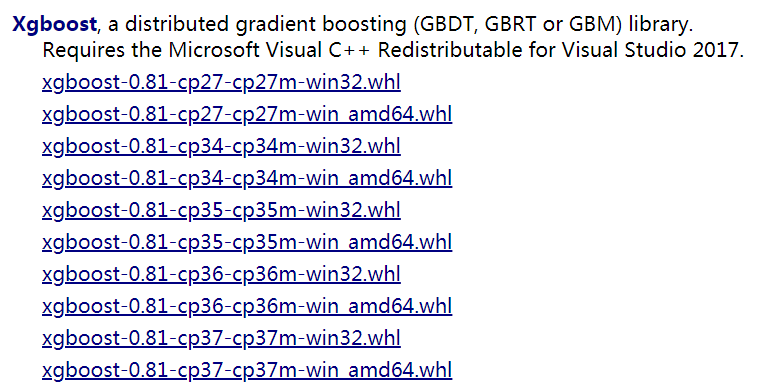2，输入安装的程式：

 1 `pip install xgboost-0.81-cp37-cp37m-win_amd64.whl`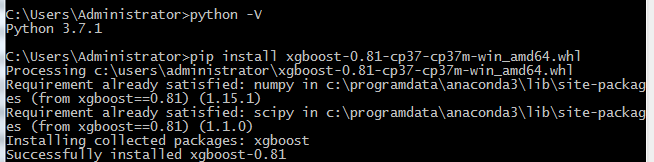## Xgboost模型详解

### 1，Xgboost能加载的各种数据格式解析

Xgboost可以加载多种数据格式的训练数据：

 1 2 3 4 5 `libsvm 格式的文本数据；` `Numpy 的二维数组；` `XGBoost 的二进制的缓存文件。加载的数据存储在对象 DMatrix 中。`

 1 `dtrain1 = xgb.DMatrix(``'train.svm.txt'``)`

 1 `dtrain2  = xgb.DMatrix(``'train.svm.buffer'``)`

 1 2 3 `data = np.random.rand(5,10)                 # 5行10列数据集` `label = np.random.randint(2,size=5)       # 二分类目标值` `dtrain = xgb.DMatrix(data,label=label)    # 组成训练集`

 1 2 `csr = scipy.sparse.csr_matrix((dat,(row,col)))` `dtrain = xgb.DMatrix( csr )`

 1 2 `dtrain = xgb.DMatrix(``'train.svm.txt'``)` `dtrain.save_binary(``"train.buffer"``)`

 1 `dtrain = xgb.DMatrix( data, label=label, missing = -999.0)`

 1 2 `w = np.random.rand(5,1)` `dtrain = xgb.DMatrix( data, label=label, missing = -999.0, weight=w)`

### 2，Xgboost的模型参数

Xgboost使用key-value字典的方式存储参数

 1 2 3 4 5 6 7 8 9 10 11 12 13 14 15 16 17 18 19 `# xgboost模型` `params` `= {` `    ``'booster'``:``'gbtree'``,` `    ``'objective'``:``'multi:softmax'``,   # 多分类问题` `    ``'num_class'``:10,  # 类别数，与multi softmax并用` `    ``'gamma'``:0.1,    # 用于控制是否后剪枝的参数，越大越保守，一般0.1 0.2的样子` `    ``'max_depth'``:12,  # 构建树的深度，越大越容易过拟合` `    ``'lambda'``:2,  # 控制模型复杂度的权重值的L2 正则化项参数，参数越大，模型越不容易过拟合` `    ``'subsample'``:0.7, # 随机采样训练样本` `    ``'colsample_bytree'``:3,# 这个参数默认为1，是每个叶子里面h的和至少是多少` `    ``# 对于正负样本不均衡时的0-1分类而言，假设h在0.01附近，min_child_weight为1` `    ``#意味着叶子节点中最少需要包含100个样本。这个参数非常影响结果，` `    ``# 控制叶子节点中二阶导的和的最小值，该参数值越小，越容易过拟合` `    ``'silent'``:0,  # 设置成1 则没有运行信息输入，最好是设置成0` `    ``'eta'``:0.007,  # 如同学习率` `    ``'seed'``:1000,` `    ``'nthread'``:7,  #CPU线程数` `    ``#'eval_metric':'auc'` `}`

Booster参数（Booster Parameters）：这取决于使用哪种booster

#### 2.1， 通用参数

booster[default = gbtree]：有两种模型可以选择gbtree 和 gblinear。gbtree使用基于树的模型进行提升计算，gblinear使用线性模型进行提升计算。缺省值为gbtree。

silent[default = 0] ：取0时表示打印出运行时信息，取1时表示已默认方式运行，不打印运行信息。缺省值为0

verbosity：[默认 =1] 打印消息的详细程度，有效值为0（静默），1（警告），2（信息），3（调试）。有时，XGBoost会尝试根据启发式更改配置，启动式显示为警告消息。如果出现意外，请尝试增加详细程度。

num_pbuffer：预测缓冲区大小，通常设置为训练实例的数目。缓冲用于保存最后一步提升的预测结果，无需人为设置。

num_feature：Boosting过程中用到的特征维数，设置为特征个数，Xgboost会自动设置，无需认为设置

disable_default_eval_metric ：[默认 = 0] 标记以禁止默认度量标准，设置为> 0 以禁止。

#### 2.2，tree booster参数

eta [default=0.3] ：为了防止过拟合，更新过程中用到的收缩步长。在每次提升计算之后，算法会直接获得新特征的权重。 eta通过缩减特征的权重使提升计算过程更加保守。缺省值为0.3

gamma [default=0] ：在节点分裂时，只有分裂后损失函数的值下降了，才会分裂这个节点。Gamma指定了节点分裂所需的最小损失函数下降值。这个参数的值越大，算法越保守。这个参数的值和损失函数息息相关，所以是需要调整的。取值范围为：[0,∞]

max_depth [default=6] ：数的最大深度。缺省值为6 。取值范围为：[1,∞]。需要使用CV函数来进行调优。典型值：3-10

min_child_weight [default=1] ：孩子节点中最小的样本权重和。如果一个叶子节点的样本权重和小于min_child_weight则拆分过程结束。在现行回归模型中，这个参数是指建立每个模型所需要的最小样本数。这个参数用于避免过拟合。当它的值较大时，可以避免模型学习到局部的特殊样本。

max_delta_step [default=0] ：我们允许每个树的权重被估计的值。如果它的值被设置为0，意味着没有约束；如果它被设置为一个正值，它能够使得更新的步骤更加保守。通常这个参数是没有必要的，但是如果在逻辑回归中类极其不平衡这时候他有可能会起到帮助作用。把它范围设置为1-10之间也许能控制更新。 取值范围为：[0,∞]

subsample [default=1] ：用于训练模型的子样本占整个样本集合的比例。如果设置为0.5则意味着XGBoost将随机的从整个样本集合中随机的抽取出50%的子样本建立树模型，这能够防止过拟合。 取值范围为：(0,1]

colsample_bytree , colsample_bylevel , colsample_bynode [默认 =1] 这是对列的子采样参数家族，所有的colsample_by* 参数的范围均为（0,1），默认值为1.

colsample_bytree [default=1] ：在建立树时对特征采样的比例。缺省值为1 。取值范围为：(0,1]

colsample_bylevel ：是每个级别的列的子采样率，对于树中达到的每个新深度级别，子采样都会发生一次，列是从当前树的列集中进行子采样的。

colsample_bynode：是每个节点（拆分）的列的子采样率。每次评估新的拆分时，都会发生一次子采样。列是从为当前级别选择的列集中进行二次采样的。

colsample_by* ：参数累积起作用。例如，与64个功能的组合将在每个拆分中留下4个功能可供选择。

 1 `{``'colsample_bytree'``:0.5, ``'colsample_bylevel'``:0.5, ``'colsample_bynode'``:0.5}`

#### 2.3，Linear Booster参数

lambda[default = 1]：L2正则的惩罚系数，增加此值可以使模型更加保守

alpha[default = 1]：L1正则的惩罚系数，增加此值可以使模型更加保守

lambda_bias：在偏置上的L2正则。缺省值为0（在L1上没有偏置项的正则，因为L1偏置时不需要）

#### 2.4，学习目标参数

objective[default = reg:linear] ：定义学习任务及相应的学习目标，可选择的目标函数如下：

• reg:linear” —— 线性回归。
• “reg:logistic”—— 逻辑回归。
• “binary:logistic”—— 二分类的逻辑回归问题，输出为概率。
• “binary:logitraw”—— 二分类的逻辑回归问题，输出的结果为wTx。
• “count:poisson”—— 计数问题的poisson回归，输出结果为poisson分布。在poisson回归中，max_delta_step的缺省值为0.7。(used to safeguard optimization)
• “multi:softmax” –让XGBoost采用softmax目标函数处理多分类问题，同时需要设置参数num_class（类别个数）
• “multi:softprob” –和softmax一样，但是输出的是ndata * nclass的向量，可以将该向量reshape成ndata行nclass列的矩阵。没行数据表示样本所属于每个类别的概率。
• “rank:pairwise” –set XGBoost to do ranking task by minimizing the pairwise loss

base_score[default = 0.5]

eval_metric[ default according to objective]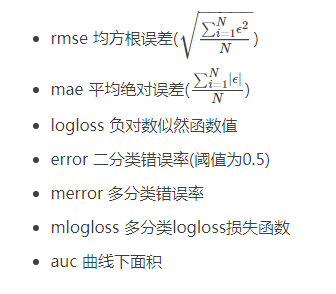seed[default=0] ：随机数的种子，缺省值为0

### 3，Xgboost基本方法和默认参数

 1 2 3 `xgboost.train(``params``,dtrain,num_boost_round=10,evals(),obj=None,` `feval=None,maximize=False,early_stopping_rounds=None,evals_result=None,` `verbose_eval=True,learning_rates=None,xgb_model=None)`

parms：这是一个字典，里面包含着训练中的参数关键字和对应的值，形式是parms = {‘booster’:’gbtree’,’eta’:0.1}

dtrain：训练的数据

num_boost_round：这是指提升迭代的个数

evals：这是一个列表，用于对训练过程中进行评估列表中的元素。形式是evals = [(dtrain,’train’),(dval,’val’)] 或者是 evals =[(dtrain,’train’)] ，对于第一种情况，它使得我们可以在训练过程中观察验证集的效果。

obj ：自定义目的函数

feval：自定义评估函数

maximize：是否对评估函数进行最大化

early_stopping_rounds：早起停止次数，假设为100，验证集的误差迭代到一定程度在100次内不能再继续降低，就停止迭代。这要求evals里至少有一个元素，如果有多个，按照最后一个去执行。返回的是最后的迭代次数（不是最好的）。如果early_stopping_rounds存在，则模型会生成三个属性，bst.best_score ,bst.best_iteration和bst.best_ntree_limit

evals_result：字典，存储在watchlist中的元素的评估结果

verbose_eval（可以输入布尔型或者数值型）：也要求evals里至少有一个元素，如果为True，则对evals中元素的评估结果会输出在结果中；如果输入数字，假设为5，则每隔5个迭代输出一次。

learning_rates：每一次提升的学习率的列表

xgb_model：在训练之前用于加载的xgb_model

### 4，模型训练

 1 2 `num_round = 10` `bst = xgb.train( plst, dtrain, num_round, evallist )`

### 5，模型预测

 1 2 3 `# X_test类型可以是二维List，也可以是numpy的数组` `dtest = DMatrix(X_test)` `ans = model.predict(dtest)`

### 6，保存模型

 1 `bst.save_model(``'test.model'``)`

 1 2 3 4 `# 导出模型到文件` `bst.dump_model(``'dump.raw.txt'``)` `# 导出模型和特征映射` `bst.dump_model(``'dump.raw.txt'``,``'featmap.txt'``)`

### 7，加载模型

 1 2 `bst = xgb.Booster({``'nthread'``:4}) # init model` `bst.load_model(``"model.bin"``)      # load data`

## Xgboost实战

Xgboost有两大类接口：Xgboost原生接口 和sklearn接口，并且Xgboost能够实现分类回归两种任务。下面对这四种情况做以解析。

### 1，基于Xgboost原生接口的分类

 1 2 3 4 5 6 7 8 9 10 11 12 13 14 15 16 17 18 19 20 21 22 23 24 25 26 27 28 29 30 31 32 33 34 35 36 37 38 39 40 41 42 43 44 45 46 47 48 49 `from` `sklearn.datasets import load_iris` `import xgboost ``as` `xgb` `from` `xgboost import plot_importance` `import matplotlib.pyplot  ``as` `plt` `from` `sklearn.model_selection import train_test_split` `from` `sklearn.metrics import accuracy_score  # 准确率` `# 记载样本数据集` `iris = load_iris()` `X,y = iris.data,iris.target` `# 数据集分割` `X_train,X_test,y_train,y_test = train_test_split(X,y,test_size=0.2,random_state=123457)` `# 算法参数` `params` `= {` `    ``'booster'``:``'gbtree'``,` `    ``'objective'``:``'multi:softmax'``,` `    ``'num_class'``:3,` `    ``'gamma'``:0.1,` `    ``'max_depth'``:6,` `    ``'lambda'``:2,` `    ``'subsample'``:0.7,` `    ``'colsample_bytree'``:0.7,` `    ``'min_child_weight'``:3,` `    ``'slient'``:1,` `    ``'eta'``:0.1,` `    ``'seed'``:1000,` `    ``'nthread'``:4,` `}` `plst = ``params``.items()` `# 生成数据集格式` `dtrain = xgb.DMatrix(X_train,y_train)` `num_rounds = 500` `# xgboost模型训练` `model = xgb.train(plst,dtrain,num_rounds)` `# 对测试集进行预测` `dtest = xgb.DMatrix(X_test)` `y_pred = model.predict(dtest)` `# 计算准确率` `accuracy = accuracy_score(y_test,y_pred)` `print(``'accuarcy:%.2f%%'``%(accuracy*100))` `# 显示重要特征` `plot_importance(model)` `plt.show()`

 1 `accuarcy:93.33%`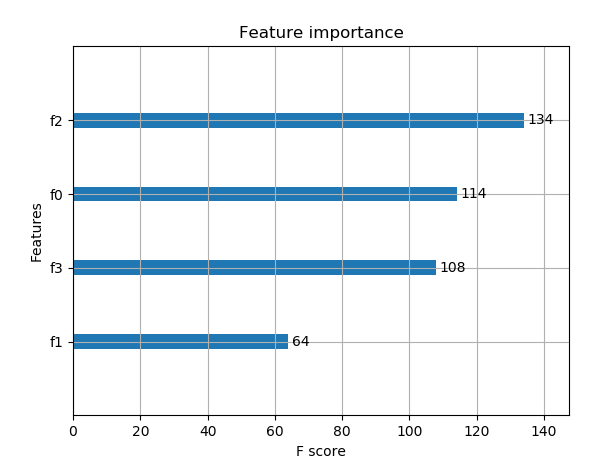### 2，基于Xgboost原生接口的回归

 1 2 3 4 5 6 7 8 9 10 11 12 13 14 15 16 17 18 19 20 21 22 23 24 25 26 27 28 29 30 31 32 33 34 35 36 37 38 39 40 41 42 `import xgboost ``as` `xgb` `from` `xgboost import plot_importance` `from` `matplotlib import pyplot ``as` `plt` `from` `sklearn.model_selection import train_test_split` `from` `sklearn.datasets import load_boston` `from` `sklearn.metrics import mean_squared_error` `# 加载数据集,此数据集时做回归的` `boston = load_boston()` `X,y = boston.data,boston.target` `# Xgboost训练过程` `X_train,X_test,y_train,y_test = train_test_split(X,y,test_size=0.2,random_state=0)` `# 算法参数` `params` `= {` `    ``'booster'``:``'gbtree'``,` `    ``'objective'``:``'reg:gamma'``,` `    ``'gamma'``:0.1,` `    ``'max_depth'``:5,` `    ``'lambda'``:3,` `    ``'subsample'``:0.7,` `    ``'colsample_bytree'``:0.7,` `    ``'min_child_weight'``:3,` `    ``'slient'``:1,` `    ``'eta'``:0.1,` `    ``'seed'``:1000,` `    ``'nthread'``:4,` `}` `dtrain = xgb.DMatrix(X_train,y_train)` `num_rounds = 300` `plst = ``params``.items()` `model = xgb.train(plst,dtrain,num_rounds)` `# 对测试集进行预测` `dtest = xgb.DMatrix(X_test)` `ans = model.predict(dtest)` `# 显示重要特征` `plot_importance(model)` `plt.show()`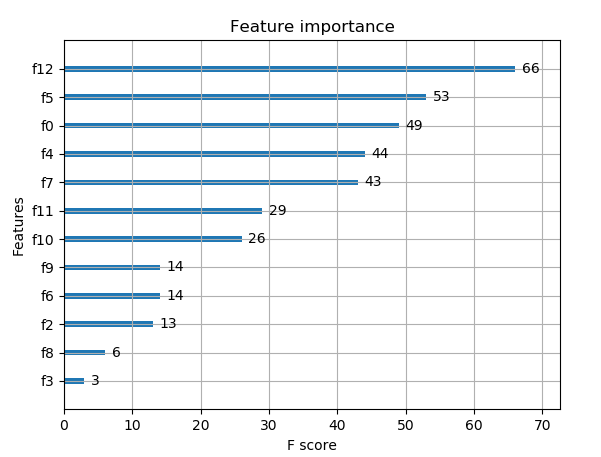### 3，Xgboost使用sklearn接口的分类（推荐）

#### XGBClassifier

 1 2 3 4 5 6 7 8 9 10 11 12 13 14 15 16 17 18 19 20 21 22 23 24 `from` `xgboost.sklearn import XGBClassifier` `clf = XGBClassifier(` `    ``silent=0,  # 设置成1则没有运行信息输出，最好是设置为0，是否在运行升级时打印消息` `    ``# nthread = 4  # CPU 线程数 默认最大` `    ``learning_rate=0.3 , # 如同学习率` `    ``min_child_weight = 1,` `    ``# 这个参数默认为1，是每个叶子里面h的和至少是多少，对正负样本不均衡时的0-1分类而言` `    ``# 假设h在0.01附近，min_child_weight为1 意味着叶子节点中最少需要包含100个样本` `    ``# 这个参数非常影响结果，控制叶子节点中二阶导的和的最小值，该参数值越小，越容易过拟合` `    ``max_depth=6, # 构建树的深度，越大越容易过拟合` `    ``gamma = 0,# 树的叶子节点上做进一步分区所需的最小损失减少，越大越保守，一般0.1 0.2这样子` `    ``subsample=1, # 随机采样训练样本，训练实例的子采样比` `    ``max_delta_step=0,  # 最大增量步长，我们允许每个树的权重估计` `    ``colsample_bytree=1, # 生成树时进行的列采样` `    ``reg_lambda=1, #控制模型复杂度的权重值的L2正则化项参数，参数越大，模型越不容易过拟合` `    ``# reg_alpha=0, # L1正则项参数` `    ``# scale_pos_weight =1 # 如果取值大于0的话，在类别样本不平衡的情况下有助于快速收敛，平衡正负权重` `    ``# objective = 'multi:softmax', # 多分类问题，指定学习任务和响应的学习目标` `    ``# num_class = 10,  # 类别数，多分类与multisoftmax并用` `    ``n_estimators=100,  # 树的个数` `    ``seed = 1000,  # 随机种子` `    ``# eval_metric ='auc'` `)`

#### 基于Sckit-learn接口的分类

 1 2 3 4 5 6 7 8 9 10 11 12 13 14 15 16 17 18 19 20 21 22 23 24 25 26 `from` `sklearn.datasets import load_iris` `import xgboost ``as` `xgb` `from` `xgboost import plot_importance` `from` `matplotlib import pyplot ``as` `plt` `from` `sklearn.model_selection import train_test_split` `from` `sklearn.metrics import accuracy_score` `# 加载样本数据集` `iris = load_iris()` `X,y = iris.data,iris.target` `X_train,X_test,y_train,y_test = train_test_split(X,y,test_size=0.2,random_state=12343)` `# 训练模型` `model = xgb.XGBClassifier(max_depth=5,learning_rate=0.1,n_estimators=160,silent=True,objective=``'multi:softmax'``)` `model.fit(X_train,y_train)` `# 对测试集进行预测` `y_pred = model.predict(X_test)` `#计算准确率` `accuracy = accuracy_score(y_test,y_pred)` `print(``'accuracy:%2.f%%'``%(accuracy*100))` `# 显示重要特征` `plot_importance(model)` `plt.show()`

 1 `accuracy:93%`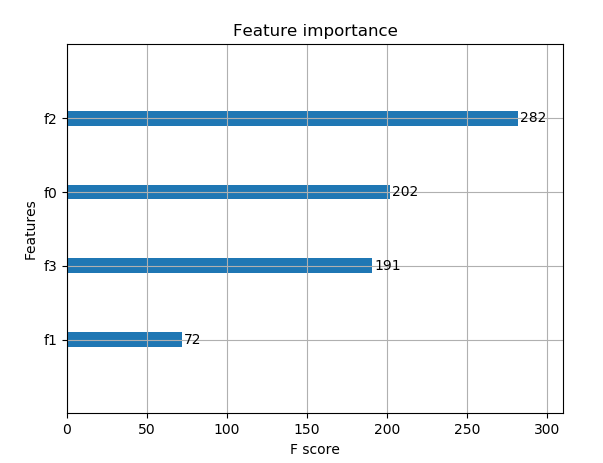### 4，基于Scikit-learn接口的回归

 1 2 3 4 5 6 7 8 9 10 11 12 13 14 15 16 17 18 19 20 21 22 `import xgboost ``as` `xgb` `from` `xgboost import plot_importance` `from` `matplotlib import pyplot ``as` `plt` `from` `sklearn.model_selection import train_test_split` `from` `sklearn.datasets import load_boston` `# 导入数据集` `boston = load_boston()` `X ,y = boston.data,boston.target` `# Xgboost训练过程` `X_train,X_test,y_train,y_test = train_test_split(X,y,test_size=0.2,random_state=0)` `model = xgb.XGBRegressor(max_depth=5,learning_rate=0.1,n_estimators=160,silent=True,objective=``'reg:gamma'``)` `model.fit(X_train,y_train)` `# 对测试集进行预测` `ans = model.predict(X_test)` `# 显示重要特征` `plot_importance(model)` `plt.show()`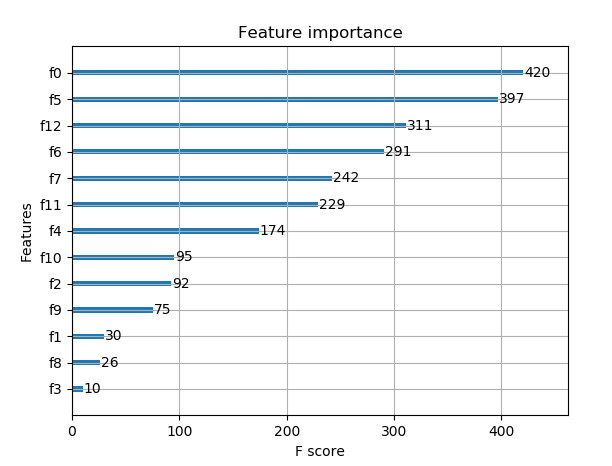## Xgboost参数调优的一般方法

### 调参步骤：

1，选择较高的学习速率（learning rate）。一般情况下，学习速率的值为0.1.但是，对于不同的问题，理想的学习速率有时候会在0.05~0.3之间波动。选择对应于此学习速率的理想决策树数量。Xgboost有一个很有用的函数“cv”，这个函数可以在每一次迭代中使用交叉验证，并返回理想的决策树数量。

2，对于给定的学习速率和决策树数量，进行决策树特定参数调优（max_depth , min_child_weight , gamma , subsample,colsample_bytree）在确定一棵树的过程中，我们可以选择不同的参数。

3，Xgboost的正则化参数的调优。（lambda , alpha）。这些参数可以降低模型的复杂度，从而提高模型的表现。

4，降低学习速率，确定理想参数。

### 第一步：确定学习速率和tree_based参数调优的估计器数目

• 1，max_depth = 5：这个参数的取值最好在3-10之间，我选的起始值为5，但是你可以选择其他的值。起始值在4-6之间都是不错的选择。
• 2，min_child_weight = 1 ：这里选择了一个比较小的值，因为这是一个极不平衡的分类问题。因此，某些叶子节点下的值会比较小。
• 3，gamma = 0 :起始值也可以选择其它比较小的值，在0.1到0.2之间就可以，这个参数后继也是要调整的。
• 4，subsample,colsample_bytree = 0.8  这个是最常见的初始值了。典型值的范围在0.5-0.9之间。
• 5，scale_pos_weight =1 这个值时因为类别十分不平衡。

### 第三步：gamma参数调优

 1 2 3 4 5 6 7 8 9 10 11 12 `param_test3 = {` ` ``'gamma'``:[i/10.0 ``for` `i ``in` `range(0,5)]` `}` `gsearch3 = GridSearchCV(estimator = XGBClassifier( learning_rate =0.1,` ` ``n_estimators=140, max_depth=4,min_child_weight=6, gamma=0,` `subsample=0.8, colsample_bytree=0.8,objective= ``'binary:logistic'``,` `nthread=4, scale_pos_weight=1,seed=27),  param_grid = param_test3, scoring=``'roc_auc'``,n_jobs=4,iid=False, cv=5)` `gsearch3.fit(train[predictors],train[target])` `gsearch3.grid_scores_, gsearch3.best_params_, gsearch3.best_score_`

## XGBoost输出特征重要性以及筛选特征

### 2，绘制特征重要性

 1 `print(model.feature_importances_)`

 1 2 3 `# plot` `pyplot.bar(range(len(model.feature_importances_)), model.feature_importances_)` `pyplot.show()`

 1 2 3 4 5 6 7 8 9 10 11 12 13 14 15 16 17 18 `# plot feature importance manually` `from` `numpy import loadtxt` `from` `xgboost import XGBClassifier` `from` `matplotlib import pyplot` `from` `sklearn.datasets import load_iris` `# load data` `dataset = load_iris()` `# split data into X and y` `X = dataset.data` `y = dataset.target` `# fit model no training data` `model = XGBClassifier()` `model.fit(X, y)` `# feature importance` `print(model.feature_importances_)` `# plot` `pyplot.bar(range(len(model.feature_importances_)), model.feature_importances_)` `pyplot.show()`

 1 `[0.17941953 0.11345647 0.41556728 0.29155672]`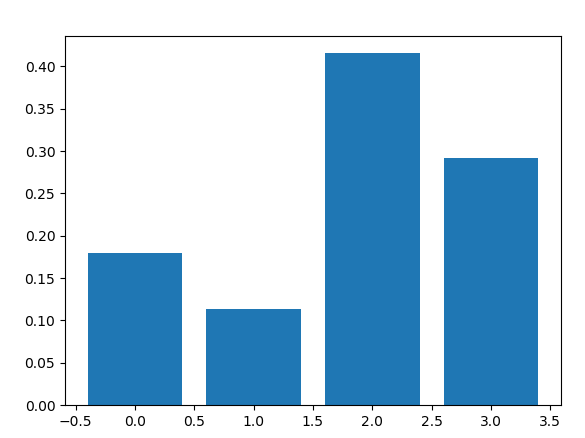1 2 3 4 5 6 7 8 9 10 11 12 13 14 15 16 17 18 19 20 21 `# plot feature importance manually` `from` `numpy import loadtxt` `from` `xgboost import XGBClassifier` `from` `matplotlib import pyplot` `from` `sklearn.datasets import load_iris` `from` `xgboost import plot_importance` `# load data` `dataset = load_iris()` `# split data into X and y` `X = dataset.data` `y = dataset.target` `# fit model no training data` `model = XGBClassifier()` `model.fit(X, y)` `# feature importance` `print(model.feature_importances_)` `# plot feature importance` `plot_importance(model)` `pyplot.show()`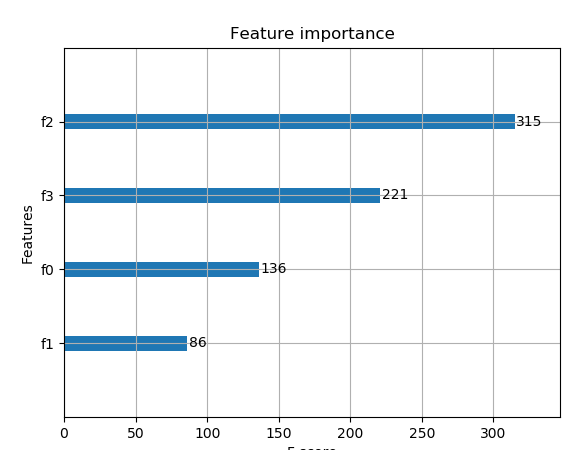### 3，根据Xgboost特征重要性得分进行特征选择

 1 2 3 4 5 6 7 8 9 `# select features using threshold` `selection = SelectFromModel(model, threshold=thresh, prefit=True)` `select_X_train = selection.transform(X_train)` `# train model` `selection_model = XGBClassifier()` `selection_model.fit(select_X_train, y_train)` `# eval model` `select_X_test = selection.transform(X_test)` `y_pred = selection_model.predict(select_X_test)`

 1 2 3 4 5 6 7 8 9 10 11 12 13 14 15 16 17 18 19 20 21 22 23 24 25 26 27 28 29 30 31 32 33 34 35 36 37 38 39 40 41 42 43 44 45 46 `# plot feature importance manually` `import numpy ``as` `np` `from` `xgboost import XGBClassifier` `from` `matplotlib import pyplot` `from` `sklearn.datasets import load_iris` `from` `xgboost import plot_importance` `from` `sklearn.model_selection import train_test_split` `from` `sklearn.metrics import accuracy_score` `from` `sklearn.feature_selection import SelectFromModel` `# load data` `dataset = load_iris()` `# split data into X and y` `X = dataset.data` `y = dataset.target` `# split data into train and test sets` `X_train,X_test,y_train,y_test = train_test_split(X,y,test_size=0.33,random_state=7)` `# fit model no training data` `model = XGBClassifier()` `model.fit(X_train, y_train)` `# feature importance` `print(model.feature_importances_)` `# make predictions for test data and evaluate` `y_pred = model.predict(X_test)` `predictions = [round(value) ``for` `value ``in` `y_pred]` `accuracy = accuracy_score(y_test,predictions)` `print(``"Accuracy:%.2f%%"``%(accuracy*100.0))` `#fit model using each importance as a threshold` `thresholds = np.sort(model.feature_importances_)` `for` `thresh ``in` `thresholds:` `    ``# select features using threshold` `    ``selection = SelectFromModel(model,threshold=thresh,prefit=True )` `    ``select_X_train = selection.transform(X_train)` `    ``# train model` `    ``selection_model = XGBClassifier()` `    ``selection_model.fit(select_X_train, y_train)` `    ``# eval model` `    ``select_X_test = selection.transform(X_test)` `    ``y_pred = selection_model.predict(select_X_test)` `    ``predictions = [round(value) ``for` `value ``in` `y_pred]` `    ``accuracy = accuracy_score(y_test,predictions)` `    ``print(``"Thresh=%.3f, n=%d, Accuracy: %.2f%%"` `% (thresh, select_X_train.shape, accuracy * 100.0))`

 1 2 3 4 5 6 `[0.20993228 0.09029345 0.54176074 0.15801354]` `Accuracy:92.00%` `Thresh=0.090, n=4, Accuracy: 92.00%` `Thresh=0.158, n=3, Accuracy: 92.00%` `Thresh=0.210, n=2, Accuracy: 86.00%` `Thresh=0.542, n=1, Accuracy: 90.00%`

https://blog.csdn.net/waitingzby/article/details/81610495

https://blog.csdn.net/u011089523/article/details/72812019

https://blog.csdn.net/luanpeng825485697/article/details/79907149